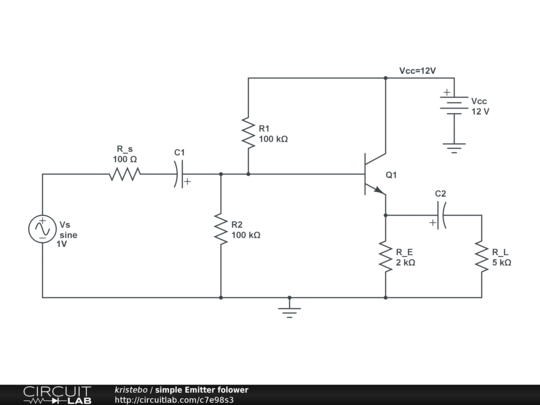# Emitter follower: Icq and Vceq calculation

I have problem with understanding how to calculate $I_{cq}$ and $V_{ceq}$ of this circuit.

Q1 has these details: $h_{FC}=150$ and $h_{fc}=100$

As I understand it I should find $V_{B}=V_{cc}(\frac{R_2}{R_1+R_2})= 6V$ then calculate $V_E=V_B-V_{BE}=5.3V$

$I_{cq} =\frac{V_E}{r_E}$ where $r_E=R_E||R_L$.

then

$V_{ceq} = V_{cc} - I_{cq} \cdot r_E$

What am I doing wrong?

Is it possible to find $V_{ceq}$ and $I_{cq}$ with the small signal parameters $h_{FC}$ and $h_{fc}$?BTW: this is NOT homework, but preparation for exams.

• For dc analysis $R_E$ is not parallel with $R_L$. $I_E$ should be 2.65mA. Everything else looks ok. Aug 13 '13 at 11:51
• that's excactly what I've found too. $I_{cq}$ and $I_E$ sould be allmost the same. Aug 13 '13 at 12:05
• @Andyaka: I have tried to do the same calculation with thevenin equivalent base voltage and resistance, should $R_{th}=R_s||R_1||R_2$ ? Aug 13 '13 at 12:12
• @Faux_Clef For DC analysis you should ignore the source branch as well, because C1 is blocking DC current there, so Rth = R1||R2
– fgp
Aug 13 '13 at 12:24
• @flpgr: of course... I did a classic "ask first, think later" Aug 13 '13 at 12:28

You can't assume the base is at 6V. The divider R1/R2 has a parallel resistance to R2 in the emitter resistance. You'll have to multiply RE with HFE to get the resistance as seen from the base. Otherwise the setting point would be independent of HFE.

Like flpgr says the emitter resistor make the circuit less dependent of HFE, but not completely. Ignoring the HFE and $R_E$ for the calculation of the base setting voltage results in a 20% error for $I_E$. You can do the calculation for different values of HFE to see how much/little HFE changes the nominal 2.2 mA.

• Do you mean I should assume that $h_{FC}=h_{FE}=\beta$ and $h_{FE} \cdot R_E = 150 \cdot 2k = 300k$ and use that as $R_E$ resistance? Aug 13 '13 at 13:02
• I get $I_E\approx \text{2.27mA}$ Aug 13 '13 at 13:07
• For a voltage divider biasing circuit RE won't make much of a difference in Vb, since hFE⋅RE is much bigger than R2. That is the whole point of this biasing circuit, to make the operating point independent of hFE as much as possible. EDIT: Didn't see that R1 and R2 were equal to 100k, thought they were 100 ohms. In this case, you need to account for Re too, you are correct.
– fgp
Aug 13 '13 at 13:15
• @Faux_Clef: Yes, the 300k is the resistance parallel to R2, so your base voltage is 5.14V. Then VE is 4.4V and IE = 4.4V/2kohm = 2.2V Aug 13 '13 at 13:19
• @flpgr: true, but you can't ignore HFE altogether. At 150 $V_B$ is 15% less than the 6V OP expected. But thereafter variations in $V_B$ due to differences in HFE should be limited. The error in $I_E$ for ignoring $V_B$ completely is 20%. Aug 13 '13 at 13:21

As Johan correctly points out, Vb won't equal exactly 6V as you have the base impedance in parallel with the lower resistor (R2)
A pretty rough calculation leaving out some finer points, for a DCcurrent gain of 150 it should be something like:

Zb = 150 * 2k = 300k

1 / (1/R2 + 1/Zb) = 75k

Vb = 12V * (75k / (75k + 100k)) = ~5.14V

Ire will be more like 2.2mA

• $I_{cq}=2.12$mA actually. It makes more sense to take impedance into account... Aug 13 '13 at 13:09

Always do the quick-and-dirty sanity check calculation first.

The current through the voltage divider is about 60 uA.

Assuming Vb = 6V, and Vbe = 0.7V, then the emitter current is ABOUT 2.65 mA.

Collector current is (beta/beta+1) x emitter current = 2.63 mA.

Corresponding base current is about 17.5 uA, which is NOT negligible compared to 60 uA, so you have to take the emitter resistor and the transistor beta into account when calculating the base voltage.

As a general rule, you want the current through the voltage divider to be AT LEAST 10x the intended base current, and more is better, because it reduces the loading from the base current on the bias divider, keeping the bias voltage closer to the intended bias.